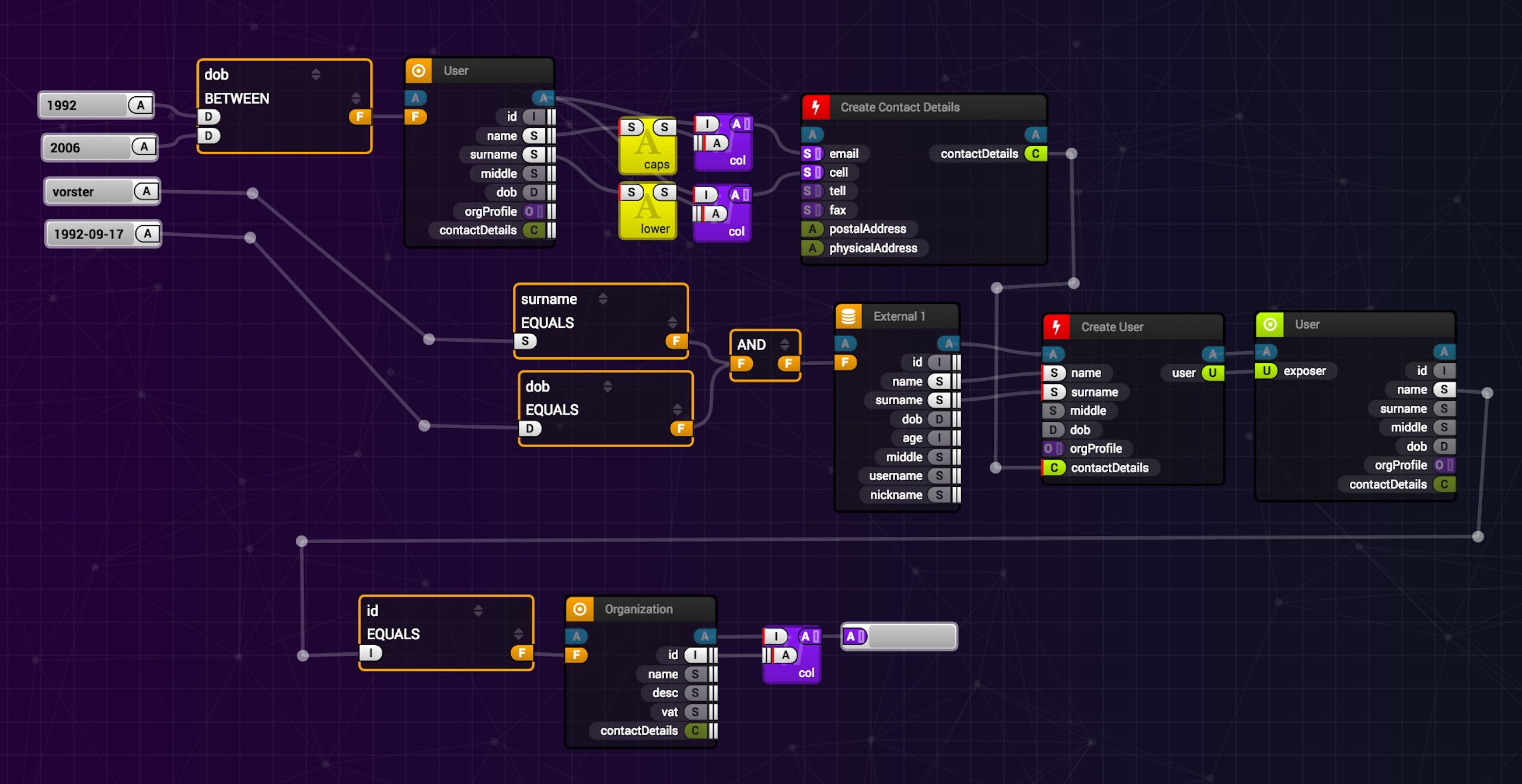## 背景

KubeFlow是业界比较具有知名度的相关开源项目：### 相关开源组件

• alibaba/GGEditor• murongqimiao/DAG-diagram• TimZaman/dagstudio• alibaba/butterfly• projectstorm/react-diagrams### 计划萌芽

React在三大前端组件库中算是生态最好的，再加上我之前有过使用经历，我仍然打算选择它。可之前写组件的经历其实不算美好，这也让我很犹豫。

• 节点
• 拖拽式创建
• 支持多选
• 可移动
• 可缩放（修改大小）
• 上下文菜单
• 自定义主题
• 输入输出端点
• 动态增删改
• 悬浮提示
• 上下文菜单
• 从输出端点拖拽创建连接边
• 从输入端点拖拽可重连接边
• 标签
• 上下文菜单
• 自定义主题
• 直线/曲线
• 画布
• 可缩放
• 适应宽度
• 适应视图
• 自定义倍数
• 可平移
• 定位到中点
• 回到原点
• 辅助对齐
• 参考线
• 支持框选
• 上下文菜单
• 自动布局
• 缩略图
• 高性能支持（10K节点）

## 前期开发

### 简单便捷的基本操作### 高性能 - 懒加载### 一些显示特效### 项目的主程序

const App: React.FC = () => {
const [flow, setFlow] = useState(genFlow()); // raw flow model
const [flowState, dispatch] = useFlowState(flow); // flow canvas state

return (
<div className='App' style={{ display: 'flex' }}>
<CanvasStyleProvider>
<FlowProvider flowState={flowState} dispatch={dispatch}>
<Canvas width='80%' height='600' />
<Toolbar>
<button onClick={() => { setFlow(genFlow()) }}>change</button>
</Toolbar>
</FlowProvider>
</CanvasStyleProvider>

<textarea readOnly style={{ width: '20%', marginTop: 48, marginLeft: 10 }} value={
'First 10 Nodes:\n' +
JSON.stringify(Object.values(flowState.raw.nodes).slice(0, 10), null, ' ')
} />
</div >
);
}

const W = 120;
const H = 40;
const Space = 10;
const RowSize = 10;
const OffsetX = 0;
const OffsetY = 48;
const NodeCount = 1000;
const EdgeCount = 50;

const generatePorts = (namePrefix: string, n: number) => {
return Array.from(Array(n).keys()).map((_, i) => ({
name: ${namePrefix}${i},
type: 'null',
}));
}

const genFlow = (): Flow => {
return {
nodes: Array.from(Array(NodeCount).keys()).reduce((o, i) => {
o[node-${i}] = { layout: { x: OffsetX + Space + (W + Space) * (i % RowSize), y: OffsetY + Space + (H + Space) * Math.trunc(i / RowSize), w: W, h: H, }, title: Component${i},
input: generatePorts("In", Math.trunc(Math.random() * 8) + 2),
output: generatePorts("Out", Math.trunc(Math.random() * 8) + 2),
};
return o;
}, {} as NodeMap),
edges: Array.from(Array(EdgeCount).keys()).reduce((o, i) => {
o[edge-${i}] = { start: { nodeId: node-${Math.trunc(Math.random() * NodeCount)},
portName: Out ${Math.trunc(Math.random() * 2)}, }, end: { nodeId: node-${Math.trunc(Math.random() * NodeCount)},
portName: In \${Math.trunc(Math.random() * 2)},
},
};
return o;
}, {} as EdgeMap),
};
}

export default App;